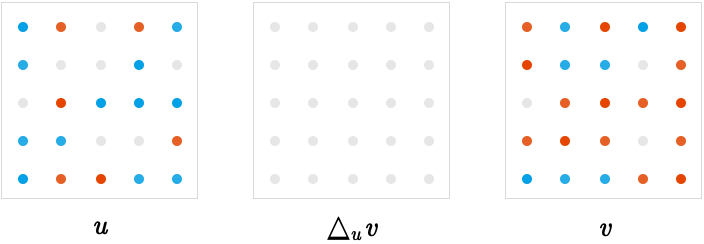$$\gdef\badDispatch#1{\textbf{\textcolor{e1432d}{#1}}} \gdef\noKatexForm#1{\badDispatch{#1}} \gdef\largeDot{\raisebox{0.06em}{\tiny∙}} \gdef\rarrbar{ {\raisebox{-0.05em}{→}\mkern{-0.13em}{\large\shortmid}}} \gdef\larrbar{ { {\large\shortmid}\mkern{-0.13em}{\raisebox{-0.05em}{←}}}} \gdef\suptrans{^\mathsf{T}} \gdef\supdagger{^\dagger} \gdef\rawsymbol#1{\operatorname{#1}} \gdef\colorsymbol#1#2{\textcolor{#1}{\rawsymbol{#2}}} \gdef\lsymbol#1{\colorsymbol{262626}{#1}} \gdef\msymbol#1{\colorsymbol{b50800}{#1}} \gdef\osymbol#1{\colorsymbol{00427f}{#1}} \gdef\lstring#1{\textcolor{666666}{\textrm{\textquotedblleft{#1}\textquotedblright}}} \gdef\boldForm#1{\textbf{#1}} \gdef\lrform#1{ {\textcolor{e1432d}{#1}}} \gdef\lgform#1{ {\textcolor{4ea82a}{#1}}} \gdef\lbform#1{ {\textcolor{3e81c3}{#1}}} \gdef\rform#1{ {\textcolor{e1432d}{#1}}} \gdef\gform#1{ {\textcolor{4ea82a}{#1}}} \gdef\bform#1{ {\textcolor{3e81c3}{#1}}} \gdef\rgform#1{ {\textcolor{dc841a}{#1}}} \gdef\gbform#1{ {\textcolor{47a5a7}{#1}}} \gdef\rbform#1{ {\textcolor{c74883}{#1}}} \gdef\waform#1{ {\textcolor{6b6b6b}{#1}}} \gdef\wbform#1{ {\textcolor{929292}{#1}}} \gdef\wcform#1{ {\textcolor{c5c5c5}{#1}}} \gdef\barToken{\mathbf{|}} \gdef\filledRectangleToken{▮} \gdef\emptyRectangleToken{▯} \gdef\filledSquareToken{■} \gdef\emptySquareToken{□} \gdef\filledToken{∙} \gdef\emptyToken{∘} \gdef\cardinalRewrite#1#2{\rewrite{#1}{#2}} \gdef\primed#1{ {#1}^{\prime}} \gdef\tinybullet{ {\tiny●}} \gdef\forwardFactor{\uparrow} \gdef\backwardFactor{\downarrow} \gdef\neutralFactor{\mid} \gdef\arrowhead{⏵} \gdef\deSymbol{ { { {\raisebox{1.1pt}{\tinybullet}\mkern{-1pt}{→}}}\,}} \gdef\ueSymbol{ {\raisebox{1pt}{\tinybullet\raisebox{-0.03em}{\mkern{-0.3pt}{\tiny──}\mkern{-0.3pt}}\tinybullet}\,}} \gdef\ldeSymbol{ { { {\raisebox{1.1pt}{\tinybullet}\mkern{-1pt}{\longrightarrow}}}\,}} \gdef\lueSymbol{ {\raisebox{1pt}{\tinybullet\raisebox{-0.03em}{\mkern{-0.3pt}{\tiny────}\mkern{-0.3pt}}\tinybullet}\,}} \gdef\de#1#2{ { {#1} \deSymbol {#2}}} \gdef\ue#1#2{ { {#1} \ueSymbol {#2}}} \gdef\tde#1#2#3{ { {#1} \overset{#3}{\deSymbol} {#2}}} \gdef\tue#1#2#3{ { {#1} \overset{#3}{\ueSymbol} {#2}}} \gdef\ltde#1#2#3{ { {#1} \overset{#3}{\ldeSymbol} {#2}}} \gdef\ltue#1#2#3{ { {#1} \overset{#3}{\lueSymbol} {#2}}} \gdef\mapsfrom{\htmlClass{hreflect}{\mapsto}} \gdef\longmapsfrom{\htmlClass{hreflect}{\longmapsto}} \gdef\diffd{𝕕} \gdef\partialdof#1{\partial {#1}} \gdef\emptySet{\empty} \gdef\unknown{\wbform{\text{?}}} \gdef\notApplicable{\wbform{\text{---}}} \gdef\textAnd{\text{\,and\,}} \gdef\identicallyEqualSymbol{\equiv} \gdef\congruentSymbol{\equiv} \gdef\isomorphicSymbol{\cong} \gdef\homotopicSymbol{\simeq} \gdef\approxEqualSymbol{\approx} \gdef\bijectiveSymbol{\cong} \gdef\defeq{\;≝\;} \gdef\defEqualSymbol{\;≝\;} \gdef\tailEqualSymbol{\underdot{=}} \gdef\headEqualSymbol{\dot{=}} \gdef\ruledelayed{:\to} \gdef\mathdollar{\text{\textdollar}} \gdef\hyphen{\text{-}} \gdef\endash{\text{--}} \gdef\emdash{\text{---}} \gdef\updownarrows{\uparrow\!\downarrow} \gdef\vthinspace{\mkern{1pt}} \gdef\dlq{\text{\textquotedblleft}} \gdef\drq{\text{\textquotedblright}} \gdef\dprime{ {\prime\prime}} \gdef\inverse#1{ { {#1}^{-1}}} \gdef\inverseSymbol{\inverse{□}} \gdef\groupDirectProduct#1{#1} \gdef\groupDirectProductSymbol{\times} \gdef\groupInverse{\inverse} \gdef\groupPower#1#2{ {#1}^{#2}} \gdef\groupCommutator#1#2{\left[{#1},{#2}\right]} \gdef\groupoidInverse{\inverse} \gdef\latticeBFS#1{\textrm{bfs}({#1})} \gdef\pathHomomorphism#1{#1} \gdef\groupoidFunction#1{#1} \gdef\groupoidHomomorphism#1{#1} \gdef\affineModifier#1{\overrightharpoon{#1}} \gdef\supercirc#1{#1^{\circ}} \gdef\supercircb#1{#1\!\degree} \gdef\toroidalModifier#1{\supercirc{#1}} \gdef\modulo#1{\supercirc{#1}} \gdef\dividesSymbol{\,|\,} \gdef\groupFunction#1{#1} \gdef\groupHomomorphism#1{#1} \gdef\automorphisms{\operatorname{Aut}} \gdef\endomorphisms{\operatorname{End}} \gdef\transportMap#1{\transportMapSymbol_{#1}} \gdef\transportMapSymbol{\tau} \gdef\action#1{#1} \gdef\selfAction#1{\hat{#1}} \gdef\actionGroupoid#1{\utilde{#1}} \gdef\cardinalGroup#1{G^*({#1})} \gdef\signed#1{ {#1}^*} \gdef\transportAtlas#1{T_{#1}} \gdef\inverted#1{\underline{#1}} \gdef\mirror#1{\overline{#1}} \gdef\pathComposeSymbol{ {\,∷\,}} \gdef\pathCompose#1#2{ {#1}\pathComposeSymbol{#2}} \gdef\translateSymbol{\uparrow} \gdef\backwardTranslateSymbol{\downarrow} \gdef\pathTranslate#1#2{ {#1}\,\translateSymbol\,{#2}} \gdef\pathLeftTranslate#1{ {#1}{\,\translateSymbol}} \gdef\pathBackwardTranslate#1#2{ {#1}{\,\backwardTranslateSymbol\,}{#2}} \gdef\pathLeftBackwardTranslate#1{ {#1}{\,\backwardTranslateSymbol}} \gdef\compactCovariantDifference#1#2{\Delta_{#1}\,{#2}} \gdef\covariantDifference{ {#1}\,\Delta\,{#2}} \gdef\forwardDifference{\Delta^{+}} \gdef\backwardDifference{\Delta^{-}} \gdef\centralDifference{\Delta} \gdef\pathForwardDifference#1{\forwardDifference_{#1}} \gdef\pathBackwardDifference#1{\backwardDifference_{#1}} \gdef\pathCentralDifference#1{\centralDifference_{#1}} \gdef\pathHead#1{\pathHeadVector{#1}} \gdef\pathTail#1{\pathTailVector{#1}} \gdef\pathHeadVector#1{ {#1}^{\bullet}} \gdef\pathTailVector#1{ {#1}_{\bullet}} \gdef\pathReverse#1{ {#1}^{\dagger}} \gdef\pathIntegral#1#2{ {#1} \int {#2}} \gdef\pathIntegralSymbol{ {\int}} \gdef\pathDot#1#2{ {#1} \cdot {#2}} \gdef\pathDotSymbol{ {\cdot}} \gdef\compactBasis#1{\mathscr{B}} \gdef\length{\operatorname{len}} \gdef\signedLength{\operatorname{len^*}} \gdef\andFn{\operatorname{and}} \gdef\orFn{\operatorname{or}} \gdef\notFn{\operatorname{not}} \gdef\vertexList{\operatorname{vertices}} \gdef\vertexList{\operatorname{vertices}} \gdef\edgeList{\operatorname{edges}} \gdef\pathList{\operatorname{paths}} \gdef\cardinalList{\operatorname{cards}} \gdef\signedCardinalList{\operatorname{cards^*}} \gdef\wordOf{\operatorname{word}} \gdef\headVertex{\operatorname{head}} \gdef\tailVertex{\operatorname{tail}} \gdef\basis{\operatorname{basis}} \gdef\split{\operatorname{split}} \gdef\lcm{\operatorname{lcm}} \gdef\minimalContractionSets{\operatorname{MCSets}} \gdef\minimalContractions{\operatorname{MC}} \gdef\grade{\operatorname{grade}} \gdef\support{\operatorname{support}} \gdef\coefficient{\operatorname{coeff}} \gdef\domain{\operatorname{domain}} \gdef\codomain{\operatorname{codomain}} \gdef\modFunction{\operatorname{mod}} \gdef\isPrime#1{#1\textrm{ prime}} \gdef\blank{\_} \gdef\emptyWord{} \gdef\multiwordSymbol#1{\mathbf{#1}} \gdef\wordSymbol#1{\mathtt{#1}} \gdef\word#1{#1} \gdef\pathMap#1{#1} \gdef\function#1{#1} \gdef\imageModifier#1{ {#1}^{\rightarrow}} \gdef\preimageModifier#1{ {#1}^{\leftarrow}} \gdef\multiImageModifier#1{ {#1}^{\Rightarrow}} \gdef\multiPreimageModifier#1{ {#1}^{\Leftarrow}} \gdef\functionComposition#1{#1} \gdef\functionCompositionSymbol{∘} \gdef\route#1#2#3{[{#1}\!:\!{#2}\!:\!{#3}]} \gdef\multiroute#1#2#3{[{#1}\!:\!{#2}\!:\!{#3}]} \gdef\pathWord#1#2#3{ {#1}\!:\!{#2}\!:\!{#3}} \gdef\nullPath{\bot} \gdef\nullElement{\bot} \gdef\path#1{#1} \gdef\vert#1{#1} \gdef\underdot#1{\underset{\raisebox{0.3em}{.}}{#1}} \gdef\tvert#1{\underdot{#1}} \gdef\hvert#1{\dot{#1}} \gdef\edge#1{#1} \gdef\card#1{\mathtt{#1}} \gdef\path#1{#1} \gdef\quiver#1{#1} \gdef\bindingRuleSymbol{\to} \gdef\compactBindingRuleSymbol{:} \gdef\cayleyQuiverSymbol#1{\selfAction{#1}} \gdef\bindCayleyQuiver#1#2{\selfAction{#1}[#2]} \gdef\bindActionQuiver#1#2{#1[#2]} \gdef\bindSize#1#2{#1(#2)} \gdef\bindCardSize#1#2{#1[#2]} \gdef\bindCards#1#2{#1[#2]} \gdef\subSize#1#2{#1_{#2}} \gdef\gridQuiver#1{\textrm{Grid}^{#1}} \gdef\treeQuiver#1{\textrm{Tree}^{#1}} \gdef\bouquetQuiver#1{\textrm{Bq}^{#1}} \gdef\lineQuiver{\textrm{L}} \gdef\cycleQuiver{\textrm{C}} \gdef\squareQuiver{\textrm{Sq}} \gdef\cubicQuiver{\textrm{Cbc}} \gdef\triangularQuiver{\textrm{Tri}} \gdef\hexagonalQuiver{\textrm{Hex}} \gdef\rhombilleQuiver{\textrm{Rmb}} \gdef\limit#1#2{\lim_{#2}\,#1} \gdef\realVectorSpace#1{\mathbb{R}^{#1}} \gdef\realVectorSpace#1{\mathbb{C}^{#1}} \gdef\matrixRing#1#2{M_{#2}(#1)} \gdef\groupoid#1{#1} \gdef\group#1{#1} \gdef\field#1{#1} \gdef\ring#1{#1} \gdef\indeterminate#1{#1} \gdef\semiring#1{#1} \gdef\sym#1{#1} \gdef\matrix#1{#1} \gdef\polynomial#1{#1} \gdef\polynomialRing#1#2{#1[{#2}]} \gdef\routeSymbol#1{#1} \gdef\multirouteSymbol#1{\mathbf{#1}} \gdef\planSymbol#1{\mathbf{#1}} \gdef\ringElement#1{#1} \gdef\matrixPart#1#2#3{#1\llbracket{#2,#3}\rrbracket} \gdef\matrixRowPart#1{#1} \gdef\matrixColumnPart#1{#1} \gdef\subMatrixPart#1#2#3{#1_{#2,#3}} \gdef\matrixDotSymbol{\cdot} \gdef\matrixPlusSymbol{+} \gdef\wordGroup#1{\wordGroupSymbol_{#1}} \gdef\wordGroupSymbol{\Omega} \gdef\wordRing#1{\wordRingSymbol_{#1}} \gdef\wordRingSymbol{\Omega\!\degree} \gdef\linearCombinationCoefficient#1{ {\textcolor{888888}{#1}}} \gdef\plan#1{#1} \gdef\planRing#1{\planRingSymbol_{#1}} \gdef\planRingSymbol{\Phi} \gdef\basisPath#1#2{\mathbf{#1}_{#2}} \gdef\basisPathWeight#1#2{ {#1}_{#2}} \gdef\unitSymbol{\mathbf{e}} \gdef\unitVertexField{\unitSymbol_1} \gdef\forwardSymbol{f} \gdef\backwardSymbol{b} \gdef\symmetricSymbol{s} \gdef\antisymmetricSymbol{a} \gdef\wordVector#1#2{\unitSymbol_{#1}^{#2}} \gdef\gradOf#1{\grad\,{#1}} \gdef\grad{\nabla} \gdef\divOf#1{\div\,{#1}} \gdef\div{\dot{\nabla}} \gdef\laplacianOf#1{\laplacian\,{#1}} \gdef\laplacian{\ddot{\nabla}} \gdef\suchThat#1#2{ {#1}|{#2}} \gdef\chart#1{\chartSymbol_{#1}} \gdef\chartSymbol{C} \gdef\graphRegionIntersectionSymbol{\cap} \gdef\graphRegionUnionSymbol{\cup} \gdef\pathIso{\simeq} \gdef\factorial#1{#1!} \gdef\power#1#2{ {#1}^{#2}} \gdef\repeatedPower#1#2{ {#1}^{#2}} \gdef\contractionLattice#1{\operatorname{Con}(#1)} \gdef\contractedRelation#1{\sim_{#1}} \gdef\isContracted#1#2{ {#1} \sim {#2}} \gdef\isContractedIn#1#2#3{ {#1} \sim_{#3} {#2}} \gdef\isNotContracted#1#2{ {#1} \not \sim {#2}} \gdef\isNotContractedIn#1#2#3{ {#1} \not \sim_{#3} {#2}} \gdef\contractionSum#1{#1} \gdef\contractionSumSymbol{\sqcup} \gdef\contractionProduct#1{#1} \gdef\contractionProductSymbol{ {\cdot}} \gdef\graph#1{#1} \gdef\graphHomomorphism#1{#1} \gdef\coversSymbol{\sqsupseteq} \gdef\coveredBySymbol{\sqsubseteq} \gdef\strictlyCoversSymbol{\sqsupset} \gdef\strictlyCoveredBySymbol{\sqsubset} \gdef\covering#1#2#3{ {#2} \sqsupseteq_{#1} {#3}} \gdef\powerSet#1{\powerSetSymbol({#1})} \gdef\powerSetSymbol{\mathscr{P}} \gdef\existsForm#1#2{\exists\,{#1}\,:\,{#2}} \gdef\forAllForm#1#2{\forall\,{#1}\,:\,{#2}} \gdef\notted#1{\notSymbol {#1}} \gdef\andSymbol{\land} \gdef\orSymbol{\lor} \gdef\notSymbol{\lnot} \gdef\latticeElement#1{#1} \gdef\latticeMeetSymbol{\wedge} \gdef\latticeJoinSymbol{\vee} \gdef\latticeTop{\top} \gdef\latticeBottom{\bot} \gdef\latticeGreaterSymbol{>} \gdef\latticeGreaterEqualSymbol{\ge} \gdef\latticeLessSymbol{<} \gdef\latticeLessEqualSymbol{\le} \gdef\grpname#1{\operatorname{\mathsf{#1}}} \gdef\mkg#1#2#3{\grpname{#1}({#2},{#3})} \gdef\mka#1#2#3{\mathfrak{#1}({#2},{#3})} \gdef\generalLinearAlgebra#1#2{\mka{gl}{#1}{#2}} \gdef\generalLinearGroup#1#2{\mkg{GL}{#1}{#2}} \gdef\specialLinearAlgebra#1#2{\mka{sl}{#1}{#2}} \gdef\specialLinearGroup#1#2{\mkg{SL}{#1}{#2}} \gdef\projectiveGeneralLinearAlgebra#1#2{\mka{pgl}{#1}{#2}} \gdef\projectiveGeneralLinearGroup#1#2{\mkg{PGL}{#1}{#2}} \gdef\projectiveSpecialLinearAlgebra#1#2{\mka{psl}{#1}{#2}} \gdef\projectiveSpecialLinearGroup#1#2{\mkg{PSL}{#1}{#2}} \gdef\orthogonalAlgebra#1#2{\mka{o}{#1}{#2}} \gdef\orthogonalGroup#1#2{\mkg{O}{#1}{#2}} \gdef\specialOrthogonalAlgebra#1#2{\mka{so}{#1}{#2}} \gdef\specialOrthogonalGroup#1#2{\mkg{SO}{#1}{#2}} \gdef\unitaryAlgebra#1{\mka{u}{#1}{#2}} \gdef\unitaryGroup#1{\mkg{U}{#1}{#2}} \gdef\specialUnitaryAlgebra#1#2{\mka{su}{#1}{#2}} \gdef\specialUnitaryGroup#1#2{\mkg{su}{#1}{#2}} \gdef\spinAlgebra#1#2{\mka{spin}{#1}{#2}} \gdef\spinGroup#1#2{\mkg{Spin}{#1}{#2}} \gdef\pinAlgebra#1#2{\mka{pin}{#1}{#2}} \gdef\pinGroup#1#2{\mkg{Pin}{#1}{#2}} \gdef\symmetricGroup#1{\grpname{Sym}({#1})} \gdef\presentation#1{#1} \gdef\groupPresentation#1#2{\left\langle\,{#1}\,\,\middle|\mathstrut\,\,{#2}\,\right\rangle} \gdef\groupRelationIso{=} \gdef\groupGenerator#1{#1} \gdef\groupRelator#1{#1} \gdef\groupElement#1{#1} \gdef\groupoidElement#1{#1} \gdef\iconstruct#1#2{ {#1}\,\,\middle|{\large\mathstrut}\,\,{#2}} \gdef\setConstructor#1#2{\left\{\,\iconstruct{#1}{#2}\,\right\}} \gdef\multisetConstructor#1#2{\left\{\mkern{-2.3pt}\left|\,\,\iconstruct{#1}{#2}\,\right|\mkern{-2.3pt}\right\}} \gdef\multisets#1{\mathscr{M}({#1})} \gdef\signedMultisets#1{\mathscr{M^*}({#1})} \gdef\setSymbol#1{#1} \gdef\multisetSymbol#1{#1} \gdef\setElementSymbol#1{#1} \gdef\multisetElementSymbol#1{#1} \gdef\multisetMultiplicitySymbol{\raisebox{.1em}{\small\#}\mkern{.1em}} \gdef\boundMultiplicityFunction#1{\operatorname{ {#1}^{\sharp}}} \gdef\constructor#1#2{\left.{#1}\,\,\middle|\mathstrut\,\,{#2}\right.} \gdef\elemOf#1#2{ { {#1} \in {#2} }} \gdef\notElemOf#1#2{ { {#1} \notin {#2} }} \gdef\edgeOf#1#2{ { {#1} {\in}_E {#2} }} \gdef\vertOf#1#2{ { {#1} {\in}_V {#2} }} \gdef\pathOf#1#2{ { {#1} {\in}_P {#2} }} \gdef\pathType#1{\operatorName{path}{#1}} \gdef\vertexType#1{\operatorName{vertex}{#1}} \gdef\edgeType#1{\operatorName{edge}{#1}} \gdef\multisetType#1{\operatorName{mset}{#1}} \gdef\signedMultisetType#1{\operatorName{mset^*}{#1}} \gdef\fromTo#1{#1} \gdef\fromToSymbol{\mapsto} \gdef\vertexCountOf#1{|{#1}|} \gdef\vertices#1{ V_{#1} } \gdef\edges#1{ E_{#1} } \gdef\vertexField#1{#1} \gdef\edgeField#1{#1} \gdef\pathVector#1{\mathbf{#1}} \gdef\pathVectorSpace#1{\mathscr{#1}} \gdef\baseField#1{#1} \gdef\finiteField#1{\mathbb{F}_{#1}} \gdef\functionSpace#1#2{#2^{#1}} \gdef\pathGroupoid#1{ { \Gamma_{#1} }} \gdef\forwardPathQuiver#1#2{ {\overrightharpoon{#1}_{#2}}} \gdef\backwardPathQuiver#1#2{ {\overrightharpoon{#1}^{#2}}} \gdef\pathQuiver#1{ {\overrightharpoon{#1}}} \gdef\mto#1#2{ {#1}\mapsto{#2}} \gdef\mtoSymbol{\mapsto} \gdef\groupWordRewriting#1{\langle{#1}\rangle} \gdef\rewrite#1#2{ {#1}\mapsto{#2}} \gdef\rewritingRule#1#2{ {#1}\mapsto{#2}} \gdef\rewritingSystem#1{\mathcal{#1}} \gdef\multiwayBFS#1{\textrm{bfs}({#1})} \gdef\rewritingStateBinding#1#2{ {\left.{#1}\!\mid\!{#2}\right.}} \gdef\rewritingRuleBinding#1#2{#1{\left[\,{#2}\,\right]}} \gdef\namedSystem#1{\mathsf{#1}} \gdef\genericRewritingSystem{\namedSystem{System}} \gdef\stringRewritingSystem{\namedSystem{Str}} \gdef\turingMachineRewritingSystem{\namedSystem{TM}} \gdef\cellularAutomatonRewritingSystem{\namedSystem{CA}} \gdef\graphRewritingSystem{\namedSystem{Gr}} \gdef\hypergraphRewritingSystem{\namedSystem{HGr}} \gdef\petriNetRewritingSystem{\namedSystem{Petri}} \gdef\ncard#1{\card{\inverted{#1}}} \gdef\mcard#1{\card{\mirror{#1}}} \gdef\nmcard#1{\card{\inverted{\mirror{#1}}}} \gdef\assocArray#1{\left\langle {#1} \right\rangle} \gdef\set#1{\{ {#1} \}} \gdef\list#1{\{ {#1} \}} \gdef\multiset#1{\lBrace {#1} \rBrace} \gdef\permutationCycle#1{#1} \gdef\permutationCycleSymbol{\to} \gdef\permutationSet#1{#1} \gdef\permutationSetSymbol{;} \gdef\transposition#1#2{ {#1} \leftrightarrow {#2}} \gdef\tuple#1{( {#1} )} \gdef\concat#1{ {#1} } \gdef\paren#1{\left( {#1} \right)} \gdef\ceiling#1{\lceil{#1}\rceil} \gdef\floor#1{\lfloor{#1}\rfloor} \gdef\translationVector#1{\left[{#1}\right]_{\textrm{T}}} \gdef\quotient#1#2{ {#1} / {#2}} \gdef\compactQuotient#1#2#3{ {#1}\;{\upharpoonright_{#2}}\;{#3}} \gdef\multilineQuotient#1#2{\frac{#1}{#2}} \gdef\idElem#1{e_{#1}} \gdef\minus#1{-{#1}} \gdef\elem{\ \in\ } \gdef\vsp{\mkern{0.4pt}} \gdef\iGmult{\vsp} \gdef\gmult{\vsp\ast\vsp} \gdef\Gmult{\vsp\ast\vsp} \gdef\gdot{\vsp\cdot\vsp} \gdef\gDot{\vsp{\,\largeDot\,}\vsp} \gdef\ellipsis{\dots} \gdef\verticalEllipsis{\vdots} \gdef\appliedRelation#1{#1} \gdef\setUnionSymbol{\cup} \gdef\setIntersectionSymbol{\cap} \gdef\setRelativeComplementSymbol{\backslash} \gdef\multisetUnionSymbol{\cup} \gdef\multisetIntersectionSymbol{\cap} \gdef\multisetRelativeComplementSymbol{\backslash} \gdef\multisetSumSymbol{+} \gdef\cartesianProductSymbol{\times} \gdef\functionSignature#1#2#3{ { {#1} : {#2} \to {#3}}} \gdef\poly#1{#1} \gdef\quiverProdPoly#1{#1} \gdef\quiverProdPower#1#2{#1^{#2}} \gdef\quiverProdTimes#1{#1} \gdef\parenLabeled#1#2{#1\ ({#2})} \gdef\parenRepeated#1#2{\parenLabeled{#1}{ {#2}\text{ times}}} \gdef\underLabeled#1#2{\underbrace{#1}_{#2}} \gdef\underRepeated#1#2{\underbrace{#1}_{#2\text{ times}}} \gdef\overLabeled#1#2{\overbrace{#1}^{#2}} \gdef\overRepeated#1#2{\overbrace{#1}^{#2\text{ times}}} \gdef\modLabeled#1#2{ {#1 }\textrm{ mod }{#2}} \gdef\freeGroup#1{F_{#1}} \gdef\cyclicGroup#1{\mathbb{Z}_{#1}} \gdef\componentSuperQuiverOfSymbol{\succ} \gdef\setCardinality#1{|{#1}|} \gdef\dependentQuiverProductSymbol{\,\times\,} \gdef\independentQuiverProductSymbol{\,⨝\,} \gdef\graphUnionSymbol{\sqcup} \gdef\graphProductSymbol{\times} \gdef\inlineProdSymbol{\mid} \gdef\serialCardSymbol{ {:}} \gdef\parallelCardSymbol{ {\mid}} \gdef\cardinalSequenceSymbol{ {:}} \gdef\cardinalProductSymbol{\inlineProdSymbol} \gdef\vertexProductSymbol{\!\inlineProdSymbol\!} \gdef\edgeProductSymbol{\inlineProdSymbol} \gdef\indexSum#1#2#3{ {\sum_{#2}^{#3} #1}} \gdef\indexProd#1#2#3{ {\prod_{#2}^{#3} #1}} \gdef\indexMax#1#2#3{ {\max_{#2}^{#3} #1}} \gdef\indexMin#1#2#3{ {\min_{#2}^{#3} #1}} \gdef\oneTo#1{1..{#1}} \gdef\zeroTo#1{0..{#1}} \gdef\qstring#1{\mathtt{"}{#1}\mathtt{"}} \gdef\qchar#1{\mathtt{'}{#1}\mathtt{'}} \gdef\lstr#1{\mathtt{#1}} \gdef\lchar#1{\mathtt{#1}} \gdef\string#1{ {#1}} \gdef\character#1{ {#1}} \gdef\homomorphismMapping#1{\assocArray{#1}} \gdef\translationPresentation#1{\textrm{Z}_{#1}} \gdef\starTranslationPresentation#1{\textrm{Z}^*_{#1}} \gdef\translationPathValuation#1{\mathcal{\overrightharpoon Z}_{#1}} \gdef\starTranslationPathValuation#1{\overrightharpoon{\mathcal{Z}^*_{#1}}} \gdef\translationWordHomomorphism#1{\mathcal{Z}_{#1}} \gdef\starTranslationWordHomomorphism#1{\mathcal{Z}^*_{#1}} \gdef\translationCardinalValuation#1{\textrm{T}_{#1}} \gdef\starTranslationCardinalValuation#1{\textrm{T}^*_{#1}}$$
Path algebra

# Path algebra

## Introduction

We are ready at this point to understand path calculus through the lens of path algebra. This will allow us to generalize the finite difference approach to operate on objects more general than vertex and edge fields, and on quivers that include complex cardinal structure that manifest the phenomenon of discrete curvature.

We previously defined $$\functionSpace{\vertices{}}{\baseField{K}}$$, the space of vertex fields, to be the space of functions from the set of vertices to some field $$K$$. These form a vector space, and in fact a ring under pointwise multiplication. The basis of this space is of course the set of vertices.

We also defined $$\functionSpace{\edges{}}{\baseField{K}}$$, the space of edge fields, similarly.

We now unify and extend these definitions via path algebra, by expressing vertices and edges as elements of a larger space: the set of finite paths.

## Path vectors

First, we notice that the set of vertices can be identified with the set of empty paths: to each vertex $$\vert{v}$$ we associate the empty path $$\idElem{\vert{v}} = \paren{\pathWord{\vert{v}}{\emptyWord{}}{\vert{v}}}$$.

What about edge fields? We could identify an edge $$\elemOf{\edge{e}}{\edges{}} = \tde{\vert{x}}{\vert{y}}{\card{c}}$$ with the 1-path $$\paren{\pathWord{\vert{x}}{\word{\card{c}}}{\vert{y}}}$$. But we must take care of orientation: edge fields are antisymmetric, in that for edge field $$\elemOf{\edgeField{h}}{\functionSpace{\edges{}}{\baseField{K}}}$$, we have $$\edgeField{h}(\inverted{\edge{e}}) = \minus{\edgeField{h}(\edge{e})}$$. Therefore we identify the edge $$\edge{e}$$ with the formal sum of paths $$\paren{\pathWord{\vert{x}}{\word{\card{c}}}{\vert{y}}} - \paren{\pathWord{\vert{y}}{\word{\inverted{\card{c}}}}{\vert{x}}}$$.

Hence we have identified the basis elements for vertex and edge fields as basis elements of a larger vector space: the vector space of all finite-length paths.

A vector of this enlarged path vector space, written $$\pathVectorSpace{P}$$, is a path vector, written $$\elemOf{\pathVector{p}}{\pathVectorSpace{P}}$$.

A path vector $$\pathVector{p}$$ is a $$\baseField{K}$$-linear combination of paths, which we will call basis paths, since they form the basis of the vector space $$\pathVectorSpace{P}$$. We can write this sum formally as $$\pathVector{p} = \indexSum{\basisPathWeight{p}{i} \, \basisPath{p}{i}}{i}{}$$, where $$\basisPath{p}{i}$$ is a basis path and $$\basisPathWeight{p}{i}$$ is the $$\baseField{K}$$-valued coefficient of the basis path in the sum, called a weight.

The set of $$\basisPath{p}{i}$$ for which $$\basisPathWeight{p}{i} \neq 0$$ will be called the support of the vector $$\pathVector{p}$$.

### Visualization

We can visualize such path vectors in a familiar way: we superimpose the paths $$\basisPath{p}{i}$$ in a single diagram, with the color indicating the weight $$\basisPathWeight{p}{i}$$ in the linear combination. Basis paths with coefficient zero (basis paths outside the support) are of course not drawn at all. For example: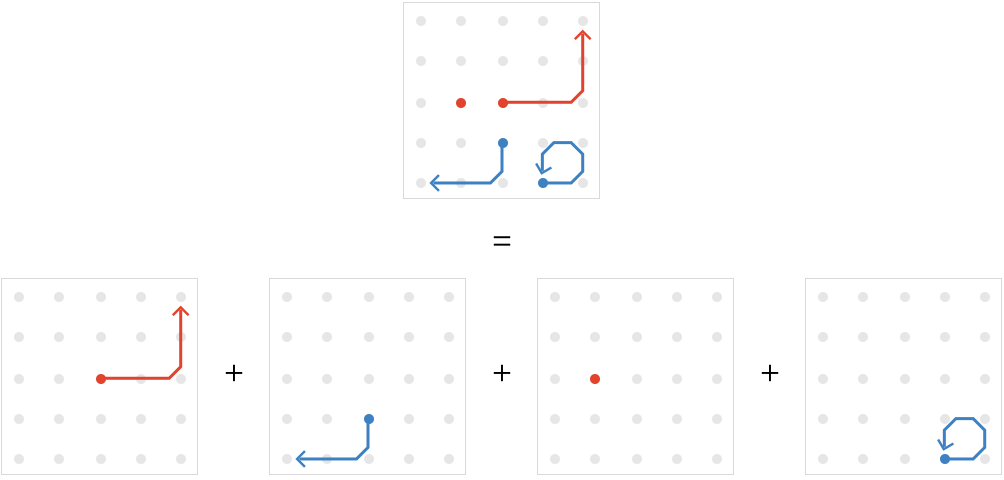We'll note that this diagramatic scheme is fully consistent with the way we have chosen to visualize vertex and edge fields in the previous section.

### Unit vertex field

The vertex fields $$\functionSpace{\vertices{}}{\baseField{K}}$$ form a vector subspace of $$\pathVectorSpace{P}$$, of course, closed under addition, multiplication, and scalar multiplication. We can identify a special vector in this subspace: $$\unitVertexField$$, the unit vertex field, with a weight 1 for every empty path:

$\unitVertexField = \indexSum{\paren{\pathWord{\vert{v}}{\emptyWord{}}{\vert{v}}}}{\elemOf{\vert{v}}{\vertices{}}}{}$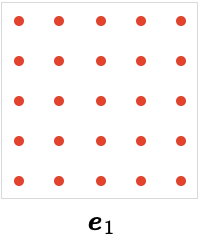### Cardinal edge fields

While the vertex fields are the simplest interesting subspace of $$\pathVectorSpace{P}$$, there is another natural subspace: the subspace of length-1 paths. There are particular vectors in these subspaces that stand out: those that are associated with a particular cardinal. The linear combination of edges, that is to say, length-1 paths, that are labeled with a given cardinal $$\card{c}$$ are called the forward cardinal edge fields, and are defined by:

$\wordVector{\word{\card{c}}}{\forwardSymbol } = \indexSum{\paren{\pathWord{\vert{t}}{\word{\card{c}}}{\vert{h}}}}{\elemOf{\vert{t},\vert{h}}{\vertices{}}}{}$

To explain this notation a bit: the summand $$\paren{\pathWord{\vert{t}}{\word{\card{c}}}{\vert{h}}}$$ is dropped if it is not a valid path, in other words, if $$\tde{\vert{t}}{\vert{h}}{\card{c}}$$ is not an edge of the quiver.

Here we show the two forward cardinal edge fields for the square lattice: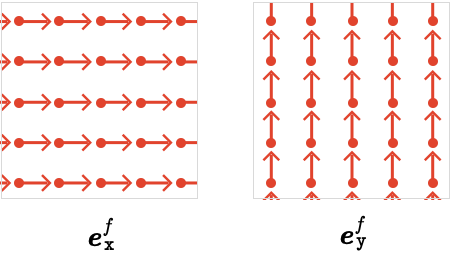We can equally well construct edge fields from the inverses of these cardinals, giving the backward cardinal edge fields:

$\wordVector{\word{\card{c}}}{\backwardSymbol } = \indexSum{\paren{\pathWord{\vert{h}}{\word{\ncard{c}}}{\vert{t}}}}{\elemOf{\vert{t},\vert{h}}{\vertices{}}}{}$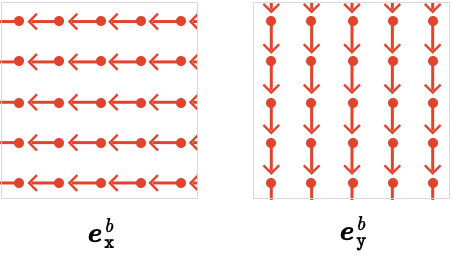The forward and backward cardinal edge fields are related via $$\wordVector{\word{\card{c}}}{\backwardSymbol } = \pathReverse{\paren{\wordVector{\word{\card{c}}}{\forwardSymbol }}}$$, where $$\pathReverse{}$$ indicates the operation of path reversal, in which we simply reverse each basis path of the vector.

A final class of cardinal edge fields are the symmetric and antisymmetric cardinal edge fields:

\begin{aligned} \wordVector{\word{\card{c}}}{\symmetricSymbol }&= \wordVector{\word{\card{c}}}{\forwardSymbol } + \wordVector{\word{\card{c}}}{\backwardSymbol }\\ \wordVector{\word{\card{c}}}{\antisymmetricSymbol }&= \wordVector{\word{\card{c}}}{\forwardSymbol } - \wordVector{\word{\card{c}}}{\backwardSymbol }\end{aligned}

Visualized for the square lattice: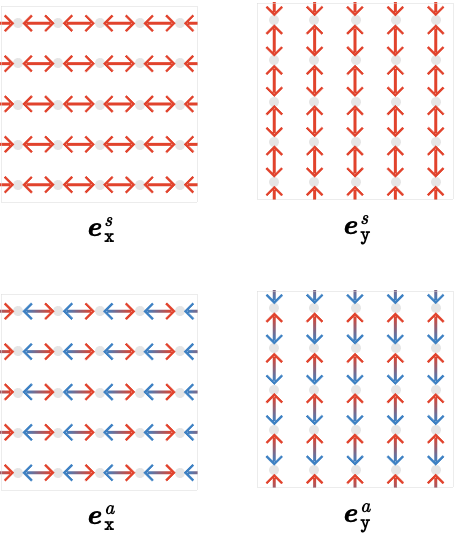We adopt a slight tweak to the visualization here: when a path and its reversal are both present, we combine them into one double-headed arrow, shading the each arrowhead to indicate the weight in that direction. This reveals the fact that the edge fields we considered in the "Path calculus" section were in fact just the antisymmetric 1-path vectors.

## Word vectors

The cardinal edge fields are themselves special cases of a more general kind of path vector called a word vector, which is the path vector which consists of the sum of all paths whose path word is $$\wordSymbol{W}$$:

zz
forward word vector$$\wordVector{\wordSymbol{W}}{\forwardSymbol }\defEqualSymbol \indexSum{\suchThat{\basisPath{p}{\sym{i}}}{\wordOf(\basisPath{p}{\sym{i}}) = \wordSymbol{W}}}{\sym{i}}{}$$
backward word vector$$\wordVector{\wordSymbol{W}}{\backwardSymbol }\defEqualSymbol \wordVector{\inverted{\wordSymbol{W}}}{\forwardSymbol } = \pathReverse{\paren{\wordVector{\wordSymbol{W}}{\forwardSymbol }}}$$
symmetric word vector$$\wordVector{\wordSymbol{W}}{\symmetricSymbol }\defEqualSymbol \wordVector{\wordSymbol{W}}{\forwardSymbol } + \wordVector{\wordSymbol{W}}{\backwardSymbol }$$
antisymmetric word vector$$\wordVector{\wordSymbol{W}}{\antisymmetricSymbol }\defEqualSymbol \wordVector{\wordSymbol{W}}{\forwardSymbol } - \wordVector{\wordSymbol{W}}{\backwardSymbol }$$

As you’d expect, if the word $$\wordSymbol{W}$$ consists of a single cardinal, we obtain the cardinal edge fields defined earlier.

Here are the word vectors corresponding to the word $$\word{\rbform{\card{x}}}{\gbform{\inverted{\card{y}}}}$$: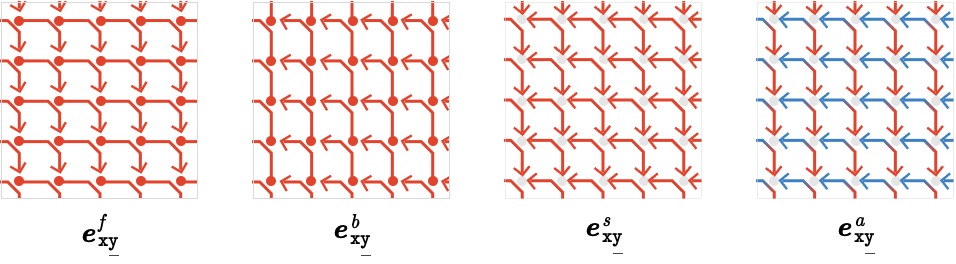As a special case, we can consider the empty word vectors, which we write with an invisible subscript. These yields the sum of empty paths, in other words, the unit vertex field $$\unitVertexField$$: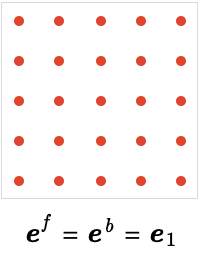We can organize this situation via the notion of a grade. The grade of an basis path $$\elemOf{\pathVector{p}}{\pathVectorSpace{P}}$$ is simply the length of the path, which we’ll write $$\grade(\pathVector{p})$$. The grade of a vector $$\pathVector{p} = \indexSum{\basisPathWeight{p}{i} \, \basisPath{p}{i}}{i}{}$$ is the single common grade of its support, if there is such a common value. If the support of $$\pathVector{p}$$ contains basis paths of different grades, we’ll say that $$\pathVector{p}$$ has mixed grade. As a special case, we consider the zero path vector $$\pathVector{0}$$ to be of undefined grade.

Since empty paths have length 0, we can now organize the situation as follows:

zzz
vertex fields$$\pathVectorSpace{P}_0$$path vectors of grade 0
edge fields$$\pathVectorSpace{P}_1$$path vectors of grade 1
$$n$$-path fields$$\pathVectorSpace{P}_{\sym{n}}$$path vectors of grade $$\sym{n}$$

Then we have the general fact that $$\pathVectorSpace{P}_{\sym{n}}$$ is a subspace of $$\pathVectorSpace{P}$$ closed under addition, multiplication, and scaling.

## Linear and bilinear operations

What is the path algebra $$\pathVectorSpace{P}\,$$good for? In other words what can we do with it, or rather, what can we do in it?

We introduce several operations, though we have seen aspects of these before:

zz
translation of $$\pathVector{p}$$ along $$\pathVector{t}$$$$\pathTranslate{\pathVector{t}}{\pathVector{p}}$$ and $$\pathBackwardTranslate{\pathVector{t}}{\pathVector{p}}$$
composition of $$\pathVector{p}$$ with $$\pathVector{q}$$$$\pathCompose{\pathVector{p}}{\pathVector{q}}$$
reversal of $$\pathVector{p}$$$$\pathReverse{\pathVector{p}}$$
head and tail of $$\pathVector{p}$$$$\pathHeadVector{\pathVector{p}}$$ and $$\pathTailVector{\pathVector{p}}$$

To define these operations formally, we need only specify what they do on the set of basis paths $$\compactBasis{\pathVectorSpace{P}}\defEqualSymbol \basis(\pathVectorSpace{P})$$. For binary operations like $$\translateSymbol$$, $$\backwardTranslateSymbol$$, and $$\pathComposeSymbol{}$$, this means defining their behavior on pairs of basis paths. For unary operations like $$\pathReverse{}$$, $$\pathHeadVector{\path{}}$$, and $$\pathTailVector{\path{}}$$, this means defining their behavior on individual basis paths. We’ll illustrate this process with specific examples, but for now, let’s define the linear-algebraic foundation of this approach.

For a unary operation $$\function{f}$$ defined on basis paths $$\functionSignature{\function{f}}{\compactBasis{\pathVectorSpace{P}}}{\compactBasis{\pathVectorSpace{P}}}$$, we define $$\boldForm{\function{f}}$$, the “vectorial” version of $$\function{f}$$, by linearity:

$\function{\boldForm{\function{f}}}(\pathVector{p})\defEqualSymbol \indexSum{\basisPathWeight{p}{\sym{i}} \, \function{f}(\basisPath{p}{\sym{i}})}{\sym{i}}{}$

If $$\function{f}$$ is a partial function not defined for certain basis paths $$\basisPath{p}{\sym{i}}$$, we define $$\function{f}(\basisPath{p}{\sym{i}}) = \pathVector{0}$$, so can simply drop such terms from the sum. This exact same construction will work if $$\function{f}$$ outputs linear combinations of basis paths, rather than single basis paths, in other words, for $$\functionSignature{\function{f}}{\compactBasis{\pathVectorSpace{P}}}{\pathVectorSpace{P}}$$.

Similarly, for a binary operation $$\function{g}$$ defined on basis paths $$\functionSignature{\function{g}}{\tuple{\compactBasis{\pathVectorSpace{P}},\compactBasis{\pathVectorSpace{P}}}}{\compactBasis{\pathVectorSpace{P}}}$$, we define vectorial $$\boldForm{\function{g}}$$ by bilinearity:

$\function{\boldForm{\function{g}}}(\pathVector{p},\pathVector{q})\defEqualSymbol \indexSum{\basisPathWeight{p}{\sym{i}} \, \basisPathWeight{q}{\sym{j}} \, \function{g}(\basisPath{p}{\sym{i}},\basisPath{q}{\sym{j}})}{\sym{i},\sym{j}}{}$

Again, if $$\function{g}$$ is a partial function that is not defined for certain pairs $$\tuple{\basisPath{p}{\sym{i}},\basisPath{q}{\sym{j}}}$$, we define $$\function{g}(\basisPath{p}{\sym{i}},\basisPath{q}{\sym{j}}) = \pathVector{0}$$. Likewise, this definition works perfectly well if $$\function{g}$$ outputs linear combinations rather than basis elements, in other words, for $$\functionSignature{\function{g}}{\tuple{\compactBasis{\pathVectorSpace{P}},\compactBasis{\pathVectorSpace{P}}}}{\pathVectorSpace{P}}$$.

### Translation

For a lattice quiver, the translation operation $$\pathTranslate{\pathVector{t}}{\pathVector{p}}$$, or “$$\pathVector{p}$$ translated along $$\pathVector{t}$$”, is defined on basis paths as follows:

$\constructor{\pathTranslate{\path{t}}{\path{p}} = \paren{\pathWord{\vert{y}}{\wordSymbol{P}}{\blank}}}{\begin{array}{c} \path{t} = \paren{\pathWord{\vert{x}}{\blank}{\vert{y}}}\\ \path{p} = \paren{\pathWord{\vert{x}}{\wordSymbol{P}}{\blank}} \end{array} }$

Here, $$\_$$ is the symbol for some vertex or cardinal, whose value is irrelevant or implied.

Put in words, $$\pathTranslate{\path{t}}{\path{p}}$$ is the path that starts where $$\path{t}\,$$ends, and has the same path word $$\wordSymbol{P}$$ as $$\path{p}$$. To be defined, $$\path{p}$$ must be “tail-to-tail” with $$\path{t}$$.

Some examples on basis paths: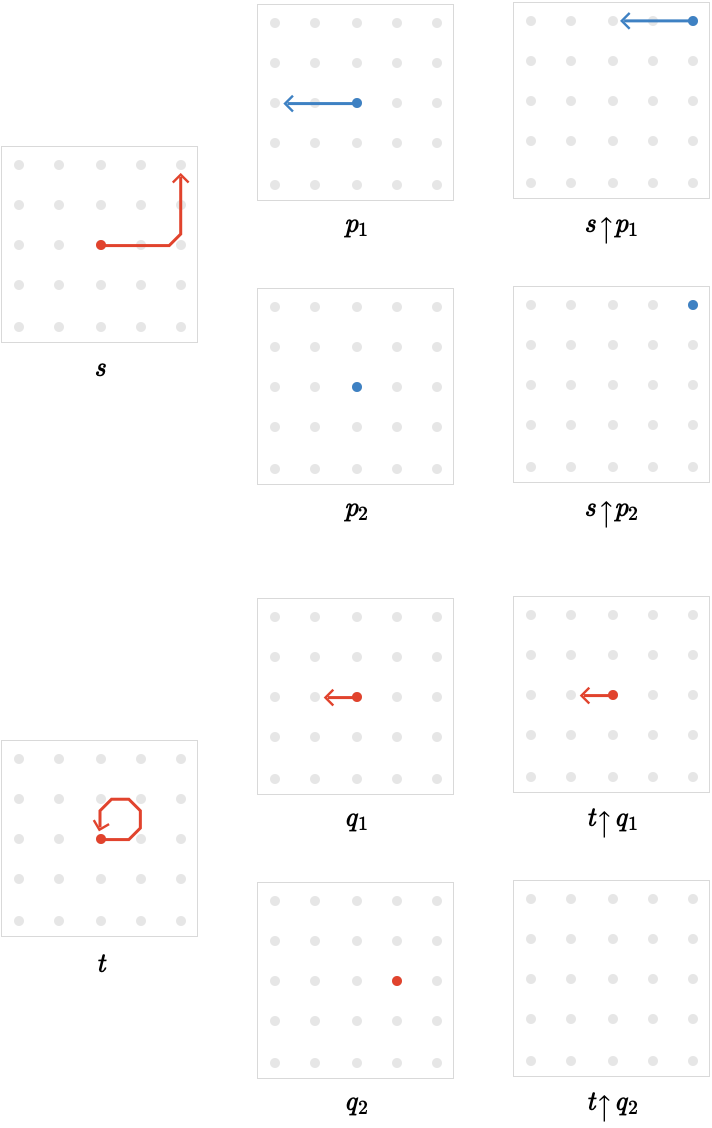The operation of translation will become considerably more subtle when move beyond perfect lattice quivers and consider the phenomenon of cardinal transport – more on that later.

### Composition

The composition operation $$\pathCompose{\pathVector{p}}{\pathVector{q}}$$, or “$$\pathVector{p}$$ composed with $$\pathVector{q}$$”, is defined on basis paths as follows:

$\constructor{\pathCompose{\path{p}}{\path{q}} = \paren{\pathWord{\vert{t}}{\concat{\wordSymbol{P} \wordSymbol{Q}}}{\vert{h}}}}{\begin{array}{c} \path{p} = \paren{\pathWord{\vert{t}}{\wordSymbol{P}}{\wbform{\vert{m}}}}\\ \path{q} = \paren{\pathWord{\wbform{\vert{m}}}{\wordSymbol{Q}}{\vert{h}}} \end{array} }$

This is just the ordinary path composition we have considered before: we can compose $$\path{p}$$ with $$\path{q}$$ if $$\path{p}$$ is “head-to-tail” with $$\path{q}$$. The result has the combined path word of $$\path{p}$$ and $$\path{q}$$.

Some examples on basis paths: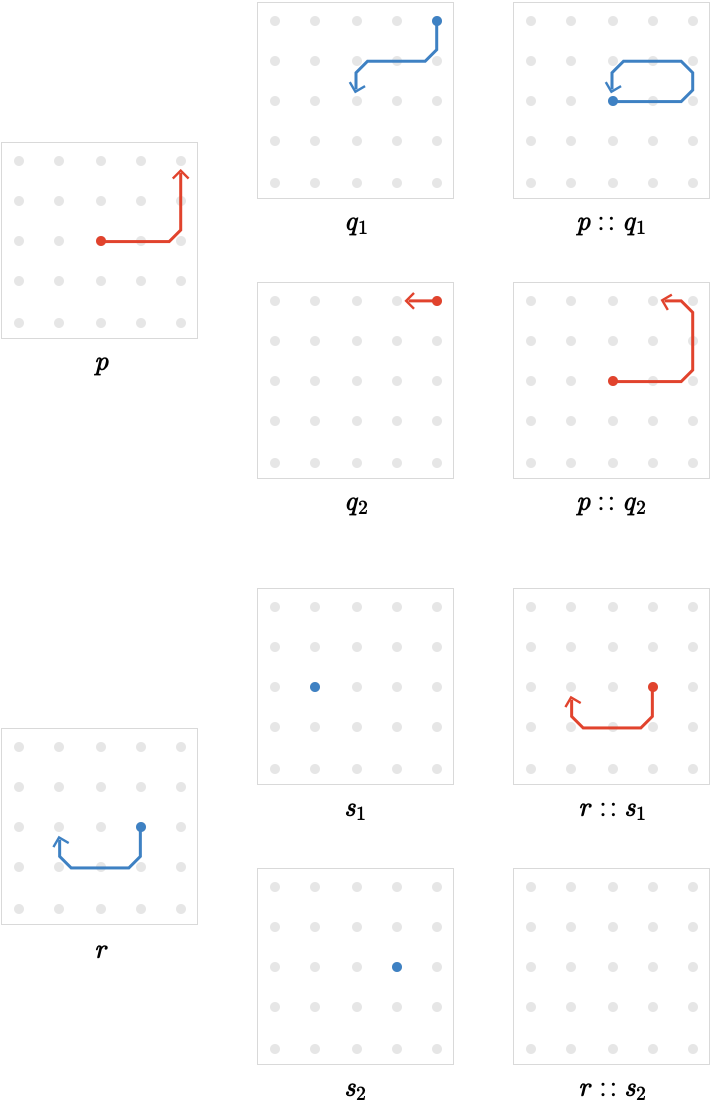### Reversal

The reversal operation $$\pathReverse{\pathVector{p}}$$ is defined on basis paths as follows:

$\constructor{\pathReverse{\path{p}} = \paren{\pathWord{\vert{y}}{\word{\ncard{\card{c}_{\sym{n}}}}{\card{\ellipsis }}{\ncard{\card{c}_2}}{\ncard{\card{c}_1}}}{\vert{x}}}}{\path{p} = \paren{\pathWord{\vert{x}}{\word{\card{\card{c}_1}}{\card{\card{c}_2}}{\card{\ellipsis }}{\card{\card{c}_{\sym{n}}}}}{\vert{y}}}}$

Or more simply, if we define the inverse of a path word to be the inversion and reversal of its constituent cardinals:

$\constructor{\pathReverse{\path{p}} = \paren{\pathWord{\vert{y}}{\inverted{\wordSymbol{W}}}{\vert{x}}}}{\path{p} = \paren{\pathWord{\vert{x}}{\wordSymbol{W}}{\vert{y}}}}$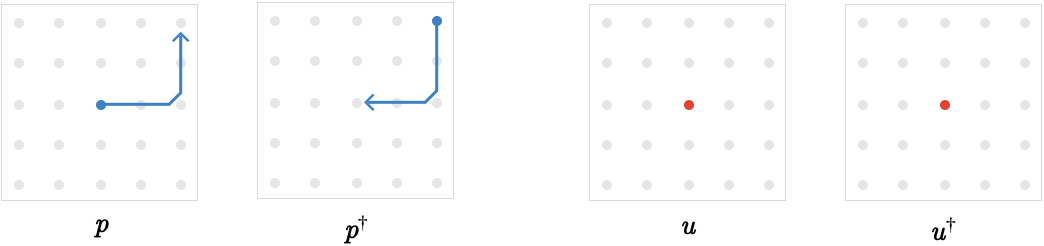The head and tail are defined as:

$\constructor{\begin{array}{c} \pathHeadVector{\path{p}} = \paren{\pathWord{\vert{y}}{\emptyWord{}}{\vert{y}}}\\ \pathTailVector{\path{p}} = \paren{\pathWord{\vert{x}}{\emptyWord{}}{\vert{x}}} \end{array} }{\path{p} = \paren{\pathWord{\vert{x}}{\blank}{\vert{y}}}}$

The head and tail operations “collapse” a path to the empty path at its head or tail vertex.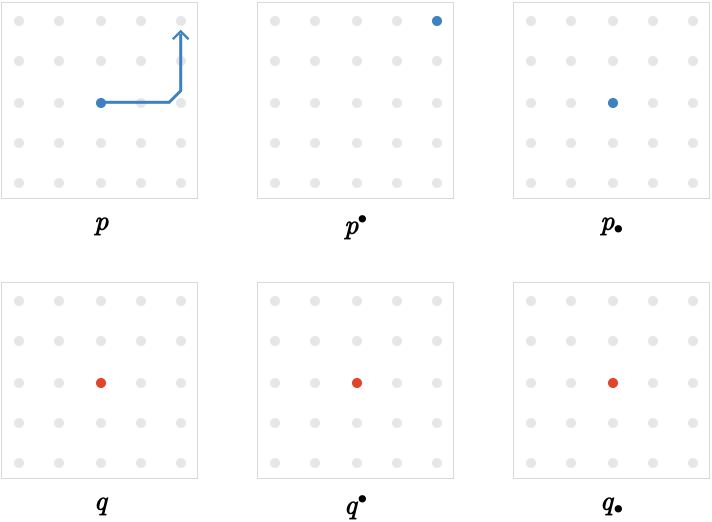## Multiset interpretation

There is a simple interpretation of the meaning of path vectors and their linear operations when we take the base field $$K$$ to be not a field but the semiring $$\mathbb{N}$$: it is the algebra of multiset operations on paths. (Technically we have now passed to a module rather than a vector space). In this picture, a path vector is a collection (a multiset) of paths, and binary operations are applied in every possible way between the two collections being operated on, with the outputs again being placed in a collection. Some of these operations may “fail”, in which case they do not contribute to the resulting collection. The coefficient of a basis path in a vector merely counts its multiplicity in the collection: how many times that path occurs in the collection.

A slightly more flexible interpretation can also be used when the base field $$K$$ is the ring $$\mathbb{Z}$$: we allow paths in the collection to "cancel" when they occur with opposite "sign". At a stretch, we can even extend this interpretation to the field $$\mathbb{Q}$$, where we can imagine sets in which multiplicities of individual basis paths are so large that we are justified in reasoning only about their multiplicity relative to some large absolute count $$N$$, neglecting the “shot noise” that occurs for small but non-zero multiplicities.

These interpretations can be a helpful guide to concrete thinking about path vectors, but of course they become nonsensical for fields like $$\mathbb{R}$$ or $$\mathbb{C}$$, although later we will advance a possible interpretation of the "meaning" of $$\mathbb{C}$$ (and its $$\mathbb{Z}$$-analog, the Gaussian integers).

## Covariant differences

Equipped with these new algebraic operations, we can generalize our previous forward, backward, and central finite differences in a straightforward way to define the covariant difference of a path vector $$Q$$ along a path vector $$P$$:

zz
forward covariant difference$$\Delta _P^+ Q=(P_ \bullet -P ) \uarr Q$$
backward covariant difference$$\Delta _P^- Q=(P^ \dag -P^ \bullet ) \uarr Q$$
central covariant difference$$\Delta _P Q=(P^ \dag -P ) \uarr Q=( \Delta _P^++ \Delta _P^- )Q$$

To avoid typesetting clutter, we’ll sometimes write $$\Delta _P Q$$ as $$P \Delta Q$$, treating $$\Delta$$ as a binary operation.

The motivation for the term covariant difference will become clearer when we consider curvature, but for now, the important aspect of these new differences is that can operate on arbitrary path vectors, not just vertex fields.

Let’s see how this works in practice. We’ll construct the forward cardinal edge fields for cardinal $$x$$ and compute the various covariant difference of an examples vertex field $$u$$.

The simplest case is the central covariant difference of a the forward edge field of $$x$$. Previously, we called this $$\Delta _x$$, which is written $$e_x^f \Delta$$ in our new notation: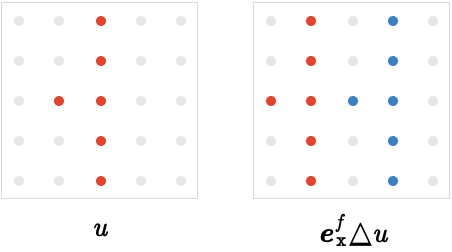The full set of combinations is shown below, with rows corresponding to the forward, backward, symmetric, and antisymmetric edge fields of $$x$$, and central three columns corresponding to the central, forward, and backward covariant differences: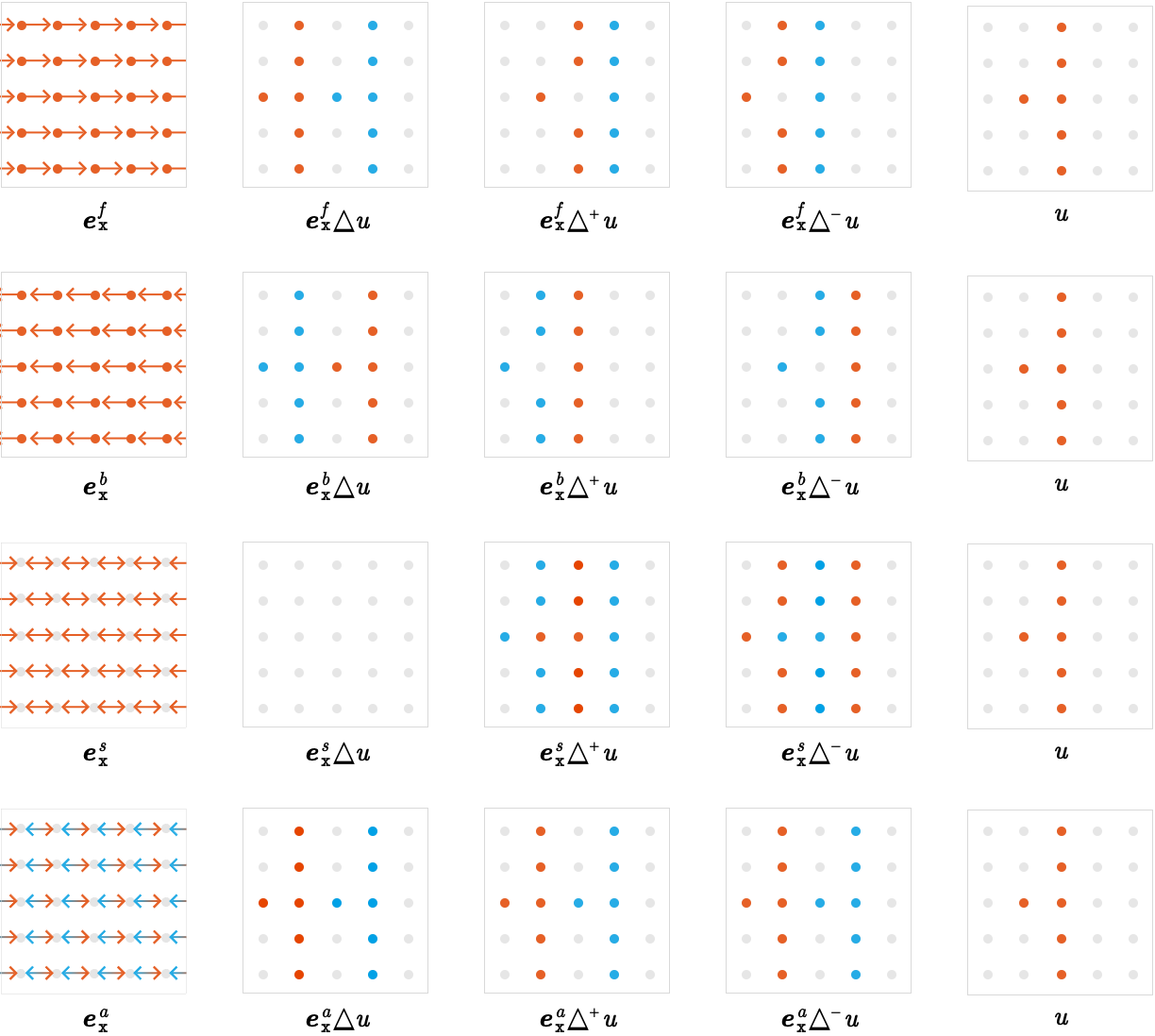We can see some identities manifested by in this table – to point them out we’ll refer to elements of this table as $$(row,column)$$.

That $$(1,3)=-(2,4)$$ and $$(1,4)=-(2,3)$$ is due to the identity $$P \Delta ^ \pm =-P^ \dag \Delta ^ \mp$$, which follows directly from the definition. That $$(1,2)=-(2,2)$$ is due to the identity $$P \Delta =-P^ \dag \Delta$$, again easily verified from the definition. That $$(3,3)=-(3,4)$$ is due to the first identity again, giving $$S \Delta ^+=-S \Delta ^-$$ for symmetric path vectors (those satisfying $$S=S^ \dag$$). That $$(3,2)=0$$ follows from the previous fact, since $$\Delta = \Delta ^++ \Delta ^-$$.

#### Differences of edge fields

What happens when we compute the covariant difference of an edge field rather than a vertex field? For perfect lattice quivers, the behavior is in some sense identical: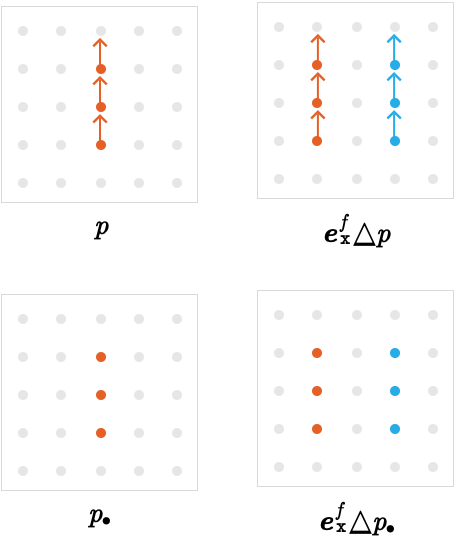The action of the difference $$e_x^f \Delta$$ on path vector $$Q$$, or in fact an arbitrary difference $$\Delta _P$$, is determined by the action of $$\Delta _P$$ on the tail vertices of $$Q$$.

We can formalize this in the following statement: $$( \Delta _PQ)_ \bullet = \Delta _P(Q_ \bullet )$$.

This is not to say that $$\Delta _PQ$$ can be recovered from $$( \Delta _PQ)_ \bullet$$, as the following example demonstrates: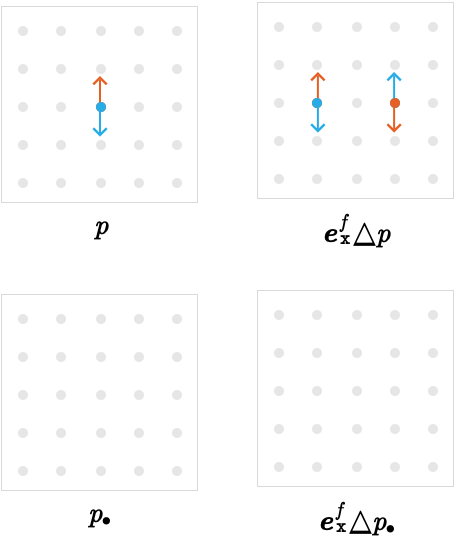Since $$p$$ consists of two 1-paths that have the same tail but opposite weight, the tail vector $$p_ \bullet$$ is zero.

We’ve seen the covariant differences taken along 1-paths, in other words, elements of $$\mathscr{P} _1$$ but what about other grades?
Since vertex fields (elements of $$\mathscr{P} _0$$) have the property that $$P=P^ \dag =P^ \bullet =P_ \bullet$$, the differences $$(P_ \bullet -P )$$ etc. used in the definition of covariant differences are identically zero, meaning the result of these covariant differences will also always be zero. We can verify this on a simple example: